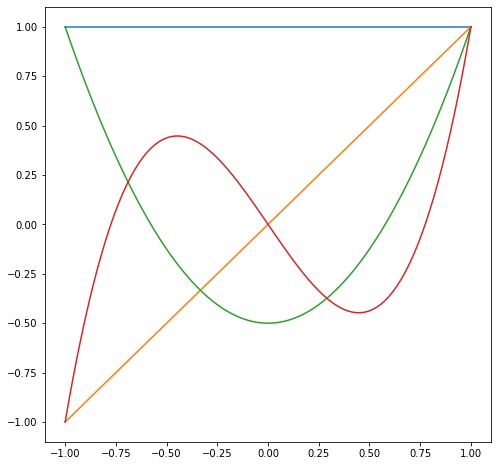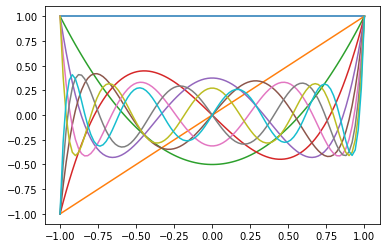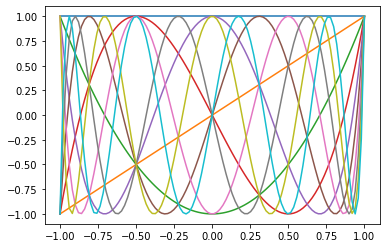# Orthogonal polynomials¶

In :
import numpy as np
import numpy.linalg as la
import matplotlib.pyplot as pt


## Mini-Introduction to sympy¶

In :
import sympy as sym

# Enable "pretty-printing" in IPython
sym.init_printing()


Make a new Symbol and work with it:

In :
x = sym.Symbol("x")

myexpr = (x**2-3)**2
myexpr

Out:
$$\left(x^{2} - 3\right)^{2}$$
In :
myexpr = (x**2-3)**2
myexpr
myexpr.expand()

Out:
$$x^{4} - 6 x^{2} + 9$$
In :
sym.integrate(myexpr, x)

Out:
$$\frac{x^{5}}{5} - 2 x^{3} + 9 x$$
In :
sym.integrate(myexpr, (x, -1, 1))

Out:
$$\frac{72}{5}$$

## Orthogonal polynomials¶

Now write a function inner_product(f, g):

In :
def inner_product(f, g):
return sym.integrate(f*g, (x, -1, 1))


Show that it works:

In :
inner_product(1, 1)

Out:
$$2$$
In :
inner_product(1, x)

Out:
$$0$$

Next, define a basis consisting of a few monomials:

In :
basis = [1, x, x**2, x**3]
#basis = [1, x, x**2, x**3, x**4, x**5]


And run Gram-Schmidt on it:

In :
orth_basis = []

for q in basis:
for prev_q in orth_basis:
q = q - inner_product(prev_q, q)*prev_q / inner_product(prev_q,prev_q)
orth_basis.append(q)

legendre_basis = [orth_basis,]

#to compute Legendre polynomials need to normalize so that q(1)=1 rather than ||q||=1
for q in orth_basis[1:]:
q = q / q.subs(x,1)
legendre_basis.append(q)

In :
legendre_basis

Out:
$$\left [ 1, \quad x, \quad \frac{3 x^{2}}{2} - \frac{1}{2}, \quad \frac{5 x^{3}}{2} - \frac{3 x}{2}\right ]$$

These are called the Legendre polynomials.

What do they look like?

In :
mesh = np.linspace(-1, 1, 100)

pt.figure(figsize=(8,8))
for f in legendre_basis:
f = sym.lambdify(x, f)
pt.plot(mesh, [f(xi) for xi in mesh])These functions are important enough to be included in scipy.special as eval_legendre:

In :
import scipy.special as sps

for i in range(10):
pt.plot(mesh, sps.eval_legendre(i, mesh))What can we find out about the conditioning of the generalized Vandermonde matrix for Legendre polynomials?

In :
#keep
n = 20
xs = np.linspace(-1, 1, n)
V = np.array([
sps.eval_legendre(i, xs)
for i in range(n)
]).T

la.cond(V)

Out:
$$7274.598185486346$$

The Chebyshev basis can similarly be defined by Gram-Schmidt, but now with respect to a different inner-product weight function, $$w(x) = 1/\sqrt{1-x^2}.$$

In :
w = 1 / sym.sqrt(1-x**2)
def cheb_inner_product(f, g):
return sym.integrate(w*f*g, (x, -1, 1))

orth_basis = []

for q in basis:
for prev_q in orth_basis:
q = q - cheb_inner_product(prev_q, q)*prev_q / cheb_inner_product(prev_q,prev_q)
orth_basis.append(q)

cheb_basis = [1,]

#to compute Legendre polynomials need to normalize so that q(1)=1 rather than ||q||=1
for q in orth_basis[1:]:
q = q / q.subs(x,1)
cheb_basis.append(q)
cheb_basis

Out:
$$\left [ 1, \quad x, \quad 2 x^{2} - 1, \quad 4 x^{3} - 3 x\right ]$$
In :
for i in range(10):
pt.plot(mesh, np.cos(i*np.arccos(mesh)))Chebyshev polynomials achieve similar good, but imperfect conditioning on a uniform grid (but perfect conditioning on a grid of Chebyshev nodes).

In :
#keep
n = 20
xs = np.linspace(-1, 1, n)
V = np.array([
np.cos(i*np.arccos(xs))
for i in range(n)
]).T

la.cond(V)

Out:
$$4846.710568240663$$